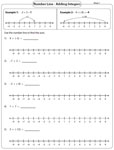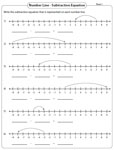Integers on Number Lines Worksheets

These worksheets contain various exercises that involve marking integers, performing addition and subtraction operation, writing addition and subtraction equations, filling the missing integers, and comparing and ordering integers on a number line.

Reading and Marking Integers

In section A, mark the integers on the number line and in section B, read each statement to mark the integers. Six exclusive sheets are here for practice.Easy:

Moderate:

Addition using number line

Use the number line to find the sum of two integers. The integers may either be positive or negative. Follow the example given in the worksheet.The addition of integers is represented on a number line. Form an addition equation for each number line. Each worksheet consists of six problems.Subtraction using number line

Find the difference of two integers using a number line. Follow the example given in the worksheets. There are five problems in each worksheet.Writing subtraction sentence

In these integers worksheets study each number line and write a subtraction equation for it.Number pattern: Number line

Understand the pattern given on each number line and fill in the missing integers. Each worksheet has seven problems.Ordering integers: Number line

Mark the given set of integers on the number line. Study the marked integers on the number line and order them from the least to the greatest.The whole batch of worksheets on integers on number lines can be downloaded instantly.